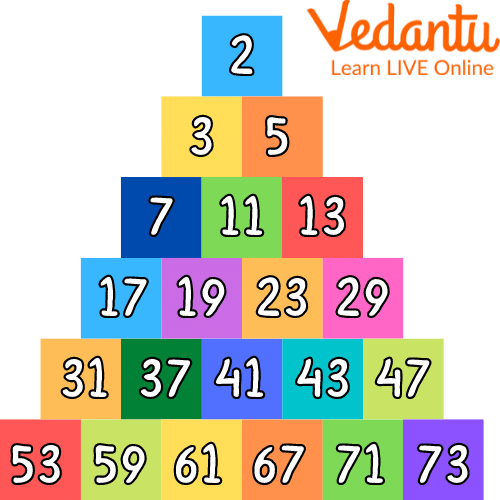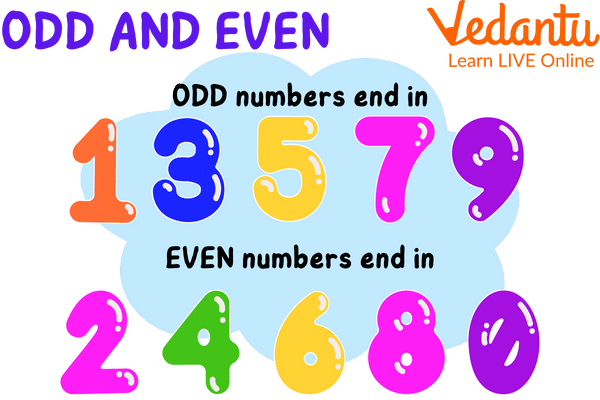Courses
Courses for Kids
Free study material
Free LIVE classes
More

# Even Composite NumbersLIVE
Join Vedantu’s FREE Mastercalss

## What is an Even Composite Number?

Any number we come across can be either a ‘prime number’ or a ‘composite number’ based upon the numbers that are able to divide the given number without leaving a remainder. These numbers are known as factors.

So depending upon the number of factors, numbers are classified as prime and composite numbers. This classification becomes important in your higher classes as you will begin to deal with factorizations and other complex operations. Now to understand what are prime and composite numbers, odd and even numbers, and the uses of understanding these core ideas, let’s jump right in!Introduction to Composite Numbers

## What is a Prime Number?

Any number that is found to have only 2 factors can be called a prime number. In other words, any number that is divisible by no other numbers other than 1 and itself can be called so. Most odd numbers are mostly prime numbers in nature.

Examples of prime numbers are 3 (divisible by 1 and 3 only), 5 (divisible by 1 and 5 only), 7 (divisible by 1 and 7 only), etc.,

2 is the only even prime number, which is an interesting fact to note as every other even number is composite numberPrime Numbers

## What is a Composite Number?

Any number that is found to have more than 2 factors can be called a composite number. In other words, any number that is divisible by numbers other than 1 and itself can be called so. Now to understand what is even composite number, most even numbers are mostly composite in nature, thus they obtain their name as even composite numbers.

Examples of composite numbers are

• 4 (divisible by 1, 4, 2)

• 6 (divisible by 1, 2, 3, 6)

• 8 (divisible by1, 2, 4, 8) and many more.Composite Numbers

## What are the Types of Composite Numbers?

Numbers in general are classified as odd numbers and even numbers, this classification holds good for composite numbers as well. Broadly composite numbers are of 2 types:

• Odd composite numbers

• Even composite numbers

Odd Composite numbers are odd numbers having more than 2 factors showing perfect divisibility. Examples of odd composite numbers are 9, 15, 21, etc.,

Odd numbers are mostly prime numbers except for a few exceptions which are odd composite numbers.

Even Composite numbers are even numbers having more than 2 factors showing perfect divisibility. Examples of even composite numbers are 4, 6, 8, 10, etc.,

Even numbers are mostly composite numbers except for 2, which is an even prime number.Odd and Even Numbers

## Solved Examples

Q 1. Check whether 213 is a composite number

Ans: A fool-proof method to find if a given number is prime or composite is by listing out the given number’s factors. 213 has the following factors 1, 213, and 71.

Since 213 has more than 2 factors, it is a composite number.

It is an odd composite number

Q 2. Check whether 187 is a composite number

Ans: 187 has the following factors 1, 187, 11 and 17

Since 187 has more than 2 factors, it is a composite number.

It is an odd composite number

Q 3. Check whether 91 is a composite number

Ans: 91 has the following factors 1, 7, 13, 91

Since 91 has more than the 2 factors, it is a composite number.

It is an odd composite number

Q 4. Check whether 78 is a composite number

Ans: 78 has the following factors 1, 2, 3, 6, 13, 39

Since 78 has more than 2 factors, it is a composite number.

It is an even composite number

Q 5. What is a composite number?

Ans: Any number that is found to have more than 2 factors can be called a composite number.

## Practice Problems

Q 1. Check whether 405 is a composite number

Ans: Yes

Q 2. Check whether 221 is a composite number

Ans: No

Q 3.____________ is the smallest composite number

Ans: 4

Q 4.___________is the smallest even prime number

Ans: 2

Q 5. Check whether 430 is a composite number

Ans: Yes

## Summary

Numbers can be classified into prime and composite numbers based on the number of factors showing perfect divisibility. These are further classified as odd and even composite and prime numbers.

When a given number has not more than 2 factors which is 1 and the number itself, then it’s called a prime number. Examples are 2, 3, 5, etc.,

When a given number has more than 2 factors which is 1 and the number itself, such a number is called a composite number. Composite numbers can be further divided into odd composite numbers and even composite numbers, Eg., 4, 6, etc.,

Last updated date: 02nd Oct 2023
Total views: 116.7k
Views today: 1.16k

## FAQs on Even Composite Numbers

1. Are all odd numbers prime and all even numbers composite in nature?

No, there are several exceptions to the above statement. First of all, most of the odd numbers are prime numbers, examples like 3, 5, 7, 11, 13, and 17….. Etc., can be seen to exhibit that common pattern. But several important examples of odd composite numbers like the numbers 9, 15, 21, 35, 49, etc., prove against the common statement.

Similarly, almost all of the even numbers are composite in nature except for 2, which is a prime number as it has two factors just 1 and the number itself

2. Is 0 and 1 composite or prime?

0 is considered to have infinite factors. Pretty much every number is a factor of zero as long as the given number is multiplied by 0. But 0 has no value, it is known as the null value. Hence it cannot come under the classification of either prime or composite number.

Similarly, 1 has only one factor which is itself. Thus it does not satisfy the definition of both the prime and composite numbers. 1 is a special number that is neither prime nor composite.

Thus both 0 and 1 are special numbers that are neither prime nor composite in nature.

3. What is the only even prime number and what are the composite numbers between 1 to 100?

The only even prime number is 2. The list of composite numbers from 1 to 100 are: 4, 6, 8, 9, 10, 12, 14, 15, 16, 18, 20, 21, 22, 24, 25, 26, 27, 28, 30, 32, 33, 34, 35, 36, 38, 39, 40, 42, 44, 45, 46, 48, 49, 50, 51,52, 54, 55, 56, 57, 58, 60, 62, 63, 64, 65, 66, 68, 69, 70, 72, 74, 75, 76, 78, 80, 81, 82, 84, 85, 86, 87, 88, 90, 91, 92, 94, 95, 96, 98, 99, 100.